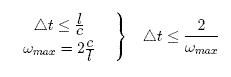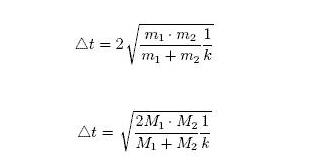# Time step size

## Wave Propagation in 3D – Continuum Wave propagation velocity in 3D-continuum: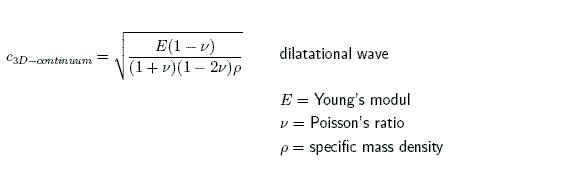comparison to rod :Critical time step :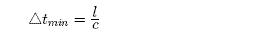comparison of critical time steps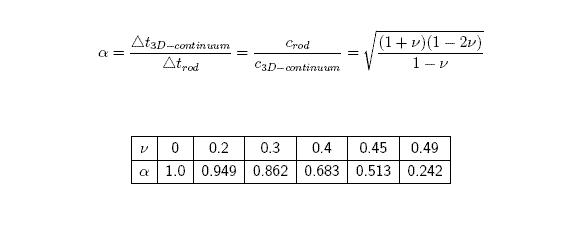materials (ν = 0.5): α –> 0

## Wave Propagation in Plane Media

Wave propagation velocity in 2D-continuum:

(twodimensional stress state)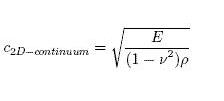comparison to rod :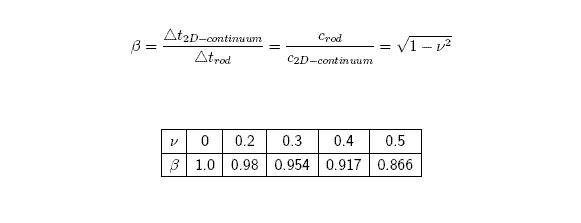• Solid elements : c 3D-continuum
• Shell elements : c 2D-continuum
• Beams & trusses : c rod

Remarks:

• The wave propagation velocity of the rod crod has the smallest value in comparison to the 2D – and 3D-continuum.
• The wave propagation velocity for membrane deformations determines the critical time step for shell and beam elements.

## Time Step Control for Beam and Truss Elements

For the Hughes-Liu beam and truss elements, the time step size is given by:where L is the length of the element and c is the sound speed:For the Belytschko beam the time step size given by the longitudinal sound speed is used, unless the bending-related time step size given by [Belytschko and Tsay 1982] governsis smaller, where I and A are the maximum value of the moment of inertia and area of the cross section, respectively.

## Characteristic length lc for Time Step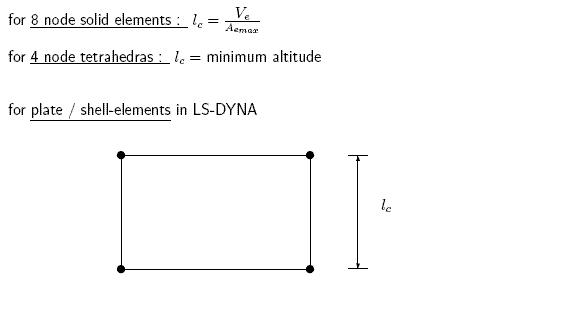warped elements :several alternatives can be selected via `*CONTROL_TIMESTEP` variable ISDO (Control Card 9, Columns 21-30), e.g.: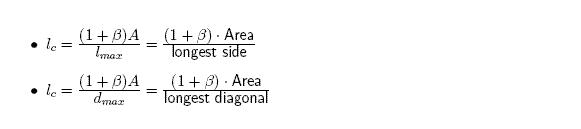where β = 0 for quadrilateral and β = 1 for triangular shell elements.

## Time Step Control for Solid Shell Elements

A critical time step size, Δ te is computed for solid shell elements fromwhere Ve is the element volume, Aemax ist the area of the largest side, and c is the plane stress sound speed## Critical Time Step for Spring Elements

Problem : There is no wave propagation velocity c to calculate critical time step size.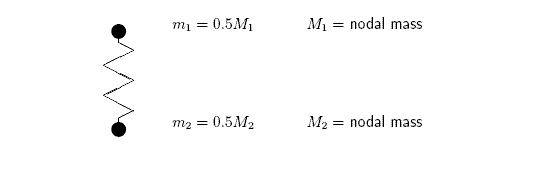Motivation : Consider free vibration of spring with nodal mass m1 and m2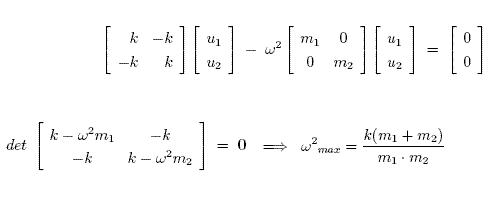Recall critical time step of rod :